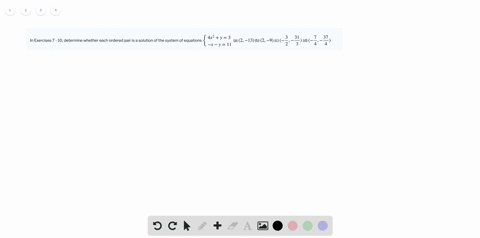Enroll in one of our FREE online STEM summer camps. Space is limited so join now!View Summer Courses### In Exercises 7 - 10, determine whether each …

02:26
CC
Kennesaw State University

Need more help? Fill out this quick form to get professional live tutoring.

Get live tutoring
Problem 7

In Exercises 7 - 10, determine whether each ordered pair is a solution of the system of equations

$\left\{\begin{array}{l} 2x - y = 4\\8x + y = -9\end{array}\right.$

(a) $(0 , -4)$
(b) $(-2 , 7)$
(c) $(\dfrac{3}{2} , -1)$
(d) $(- \dfrac{1}{2} , -5)$

a) $(D)(-1 / 2,-5)$
b) $(D)(-1 / 2,-5)$
c) $(D)(-1 / 2,-5)$
d) $(D)(-1 / 2,-5)$

## Discussion

You must be signed in to discuss.